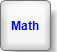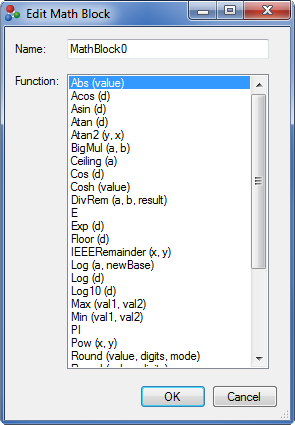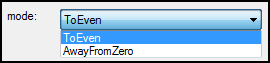← Back to X_TRADER® Help Library

#### Math Block

##### Operations

The Math Block provides the user methods for trigonometric, logarithmic, and other common mathematical functions.

When the user selects a function from the drop-down menu the Math Block exposes the corresponding number of input ports and output ports. For example, if the user selects Log (a, newBase), then the Math Block exposes two input ports and one output port.View: Double-Click Edit Window

##### Double-Click Edit Window

Double-click on the block to bring up an edit window:• For Round (value, mode) and Round (value, digits, mode) a drop-down menu box displays below the function. This allows the user to specify how to round the value if it is midway between two other numbers.View: Math Block Functions

##### Math Block Functions
Function Description
Abs(value) Returns the absolute value of the specified number.
Acos (d) Returns the angle whose cosine is the specified number.
Asin (d) Returns the angle whose sine is the specified number.
Atan (d) Returns the angle whose tangent is the specified number.
Atan2 (y,x) Returns the angle whose tangent is the quotient of two specified numbers.
BigMul (a, b) Produces the full product of two numbers.
Ceiling(a) Returns the smallest integral value that is greater than or equal to the specified decimal number.
Cos (a) Returns the cosine of the specified angle.
Cosh (value) Returns the hyperbolic cosine of the specified angle.
DivRem(a, b, result) Calculates the quotient of two integers and also returns the remainder in an output parameter.
E Represents the natural logarithmic base, specified by the constant, e.
Exp (d) Returns e raised to the specified power.
Floor(d) Returns the largest integer less than or equal to the specified decimal number.
IEEERemainder (x, y) Returns the remainder resulting from the division of a specified number by another specified number.
Log (a, newbase) Returns the logarithm of a specified number in a specified base.
Log (d)

Returns the natural (base e) logarithm of a specified number.

Log10 (d) Returns the base 10 logarithm of a specified number.
Max (val1, val2) Returns the larger of two integers.
Min (val1, val2) Returns the smaller of two integers.
PI Represents the ratio of the circumference of a circle to its diameter, specified by the constant, π.
Pow (x, y) Returns a specified number raised to the specified power.
Round (value, digits, mode) Rounds a decimal value to a specified number of fractional digits. A parameter specifies how to round the value if it is midway between two other numbers.
Round (value, digits) Rounds a decimal value to a specified number of fractional digits.
Round (a) Rounds a decimal value to the nearest integral value.
Round (value, mode) Rounds a decimal value to the nearest integer. A parameter specifies how to round the value if it is midway between two other numbers.
Sign(value) Returns a value indicating the sign of a decimal number.
Sin (a) Returns the sine of the specified angle.
Sinh (value) Returns the hyperbolic sine of the specified angle.
Sqrt (d) Returns the square root of a specified number.
Tan (a) Returns the tangent of the specified angle.
Tanh (value) Returns the hyperbolic tangent of the specified angle.
Truncate(d) Calculates the integral part of a specified decimal number.